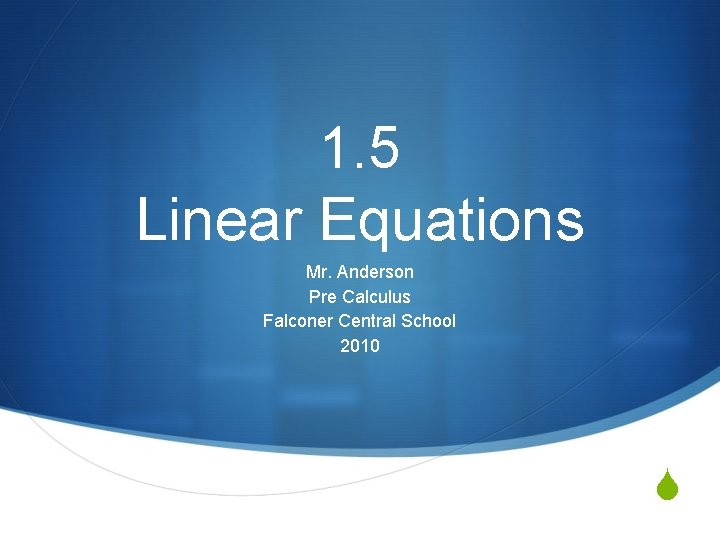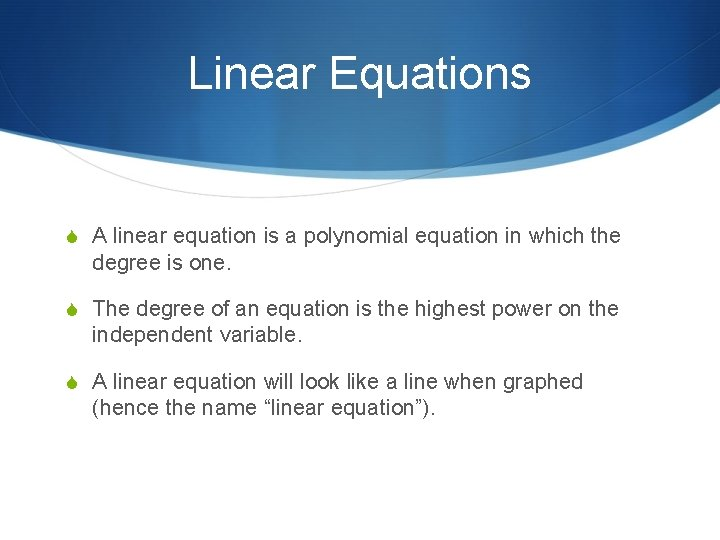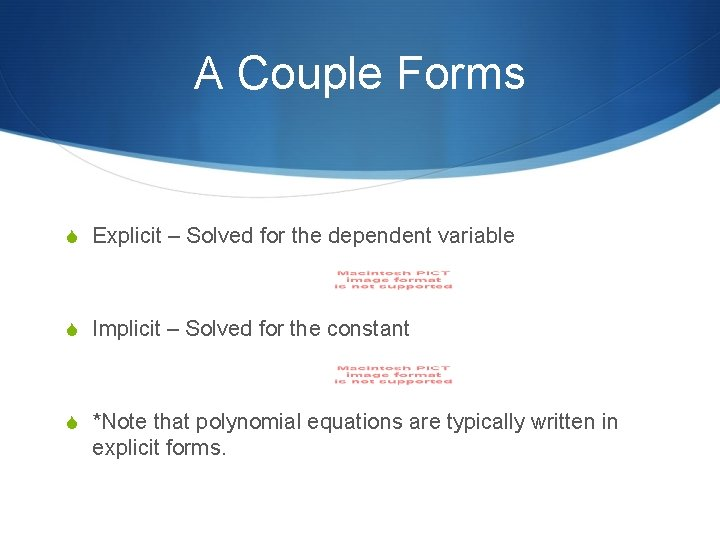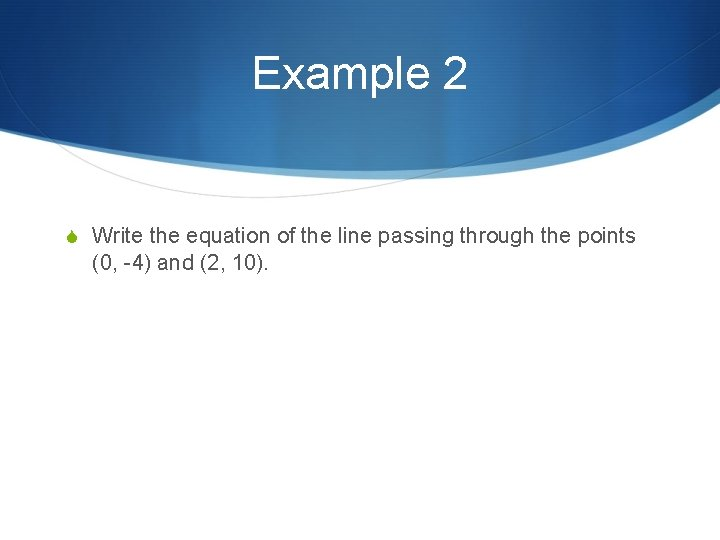# 1 5 Linear Equations Mr Anderson Pre Calculus

• Slides: 91. 5 Linear Equations Mr. Anderson Pre Calculus Falconer Central School 2010 SLinear Equations S A linear equation is a polynomial equation in which the degree is one. S The degree of an equation is the highest power on the independent variable. S A linear equation will look like a line when graphed (hence the name “linear equation”).A Couple Forms S Explicit – Solved for the dependent variable S Implicit – Solved for the constant S *Note that polynomial equations are typically written in explicit forms.Slope – Intercept Form S This is the form that we are used to. It requires a slope, and a y-intercept (hence the name).Point-Slope Form S This form is useful when you know a point and a slope (hence the name).Example 1 S Write the equation of the line passing through the point (2, 3) and having a slope of -2. orExample 2 S Write the equation of the line passing through the points (0, -4) and (2, 10).Example 3 S Suppose that in the Creative Design model of the Universe, the designer got lazy and decided that the height to weight ratio of all animals should be linear. That is, a dog that measures 1 – foot in height would weigh 14 pounds, and an elephant that is 11 feet tall would weigh 8000 pounds. In this linear model, how much would a 6 foot tall person weigh?Practice S Pg. 40 # 15 -20 , 21 -26 , 35